Question

For copper, Cu, the heat of fusion at its normal melting point of 1083 °C is...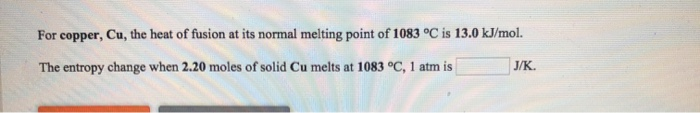For copper, Cu, the heat of fusion at its normal melting point of 1083 °C is 13.0 kJ/mol. The entropy change when 2.20 moles of solid Cu melts at 1083 °C, 1 atm is J/K

We need at least 10 more requests to produce the answer.

0 / 10 have requested this problem solution

The more requests, the faster the answer.

All students who have requested the answer will be notified once they are available.

Earn Coins

Coins can be redeemed for fabulous gifts.

Similar Homework Help Questions
• 14. For bismuth, Bi, the heat of fusion at its normal melting point of 271 °C...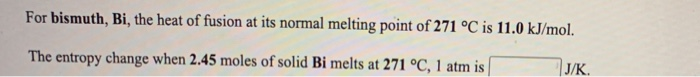14. For bismuth, Bi, the heat of fusion at its normal melting point of 271 °C is 11.0 kJ/mol. The entropy change when 2.45 moles of solid Bi melts at 271 °C, 1 atm is J/K. For magnesium, Mg, the heat of fusion at its normal melting point of 649 °C is 9.0 kJ/mol. The entropy change when 1.72 moles of liquid Mg freezes at 649 °C, 1 atm is J/K

• For ethanol, C2H5OH, the heat of fusion at its normal melting point of -115 °C is...

For ethanol, C2H5OH, the heat of fusion at its normal melting point of -115 °C is 5.0 kJ/mol. The entropy change when 1.84 moles of solid C2H5OH melts at -115 °C, 1 atm is J/K.

• The enthalpy of fusion of cadmium at its normal melting point of 321 °C is 6.11...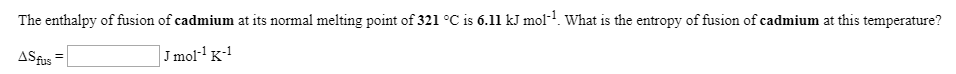The enthalpy of fusion of cadmium at its normal melting point of 321 °C is 6.11 kJ mol? What is the entropy of fusion of cadmium at this temperature? ASfus = J mol-K-1 The molar enthalpy of fusion of solid cadmium is 6.11 kJ mol-1, and the molar entropy of fusion is 10.3 JK+mol-1. (a) Calculate the Gibbs free energy change for the melting of 1.00 mol of cadmium at 622 K. (b) Calculate the Gibbs free energy change for...

• The enthalpy of fusion of bismuth at its normal melting point of 271 °C is 11.0...

The enthalpy of fusion of bismuth at its normal melting point of 271 °C is 11.0 kJ mol-1. What is the entropy of fusion of bismuth at this temperature? ΔSfus =  J mol-1 K-1

• For bismuth, Bi, the heat of vaporization at its normal boiling point of 1627 °C is...

For bismuth, Bi, the heat of vaporization at its normal boiling point of 1627 °C is 172.0 kJ/mol. The entropy change when 2.11 moles of Bi vapor condenses at 1627 °C, 1 atm is J/K.

• For silicon, Si, the heat of vaporization at its normal boiling point of 2355 °C is...

For silicon, Si, the heat of vaporization at its normal boiling point of 2355 °C is 297.1 kJ/mol. The entropy change when 2.26 moles of Si vapor condenses at 2355 °C, 1 atm is J/K.

• For cobalt, Co, the heat of vaporization at its normal boiling point of 3097 °C is...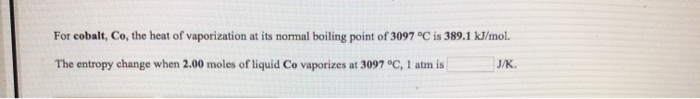For cobalt, Co, the heat of vaporization at its normal boiling point of 3097 °C is 389.1 kJ/mol. The entropy change when 2.00 moles of liquid Co vaporizes at 3097 °C, 1 atm is J/K

• 6. The melting point of CaTiSiOs is 1400°C and the heat of fusion at the normal melting point is 123 700 J mol-1. Calculate the heat of fusion at 1300°C. Cnsolid = 177.4 + 23.2 x 10', 40.3 x...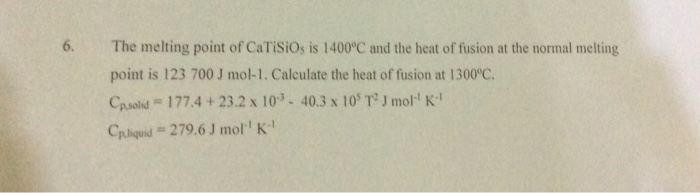6. The melting point of CaTiSiOs is 1400°C and the heat of fusion at the normal melting point is 123 700 J mol-1. Calculate the heat of fusion at 1300°C. Cnsolid = 177.4 + 23.2 x 10', 40.3 x 105 T2 J mor' K" Cphquid # 279.6 J mol'' K" 6. The melting point of CaTiSiOs is 1400°C and the heat of fusion at the normal melting point is 123 700 J mol-1. Calculate the heat of fusion at 1300°C....

• The enthalpy of fusion of manganese at its normal melting point of 1244 ? is 14.6...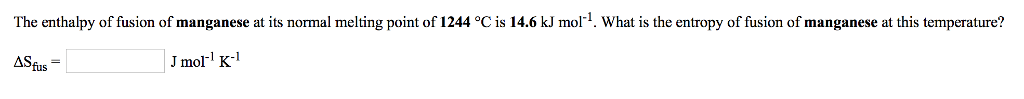The enthalpy of fusion of manganese at its normal melting point of 1244 ? is 14.6 kJ mol-1 What is the entropy of fusion of manganese at this temperature? ASfus Jmol K

• The heat of melting for Cu is 13,260 J/mol and the equilibrium melting temperature of Cu...

The heat of melting for Cu is 13,260 J/mol and the equilibrium melting temperature of Cu is 1358 K. Assuming the constant pressure heat capacities of solid Cu and liquid Cu are the same, 24.0 J/(mol K) What is the entropy change for the surroundings, which is also at 1000 K, during solidification from one mole of liquid Cu to one mole solid Cu in the system at 1000 K?